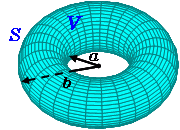# Surface Area and Volume of Torus Calculation#Torus Calculator

An online torus calculator to find the surface area and volume.

m
m# Surface Area and Volume of Torus FormulaTorus Surface Area Equation

S = π2 * (R2 - r2)

Torus Volume Equation

V = π2 * (R + r) * (R - r)2Related Calculators
Distance Between Two Points
Distance Between Two MidPoints
Short Distance Between Two Lines

##Online math number calculation, formulas ►

Online Algebra calculation, formulas , Digital calculation, Statistical calculation, Math Converters Pet Age Calculator,